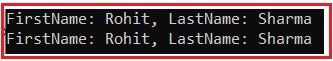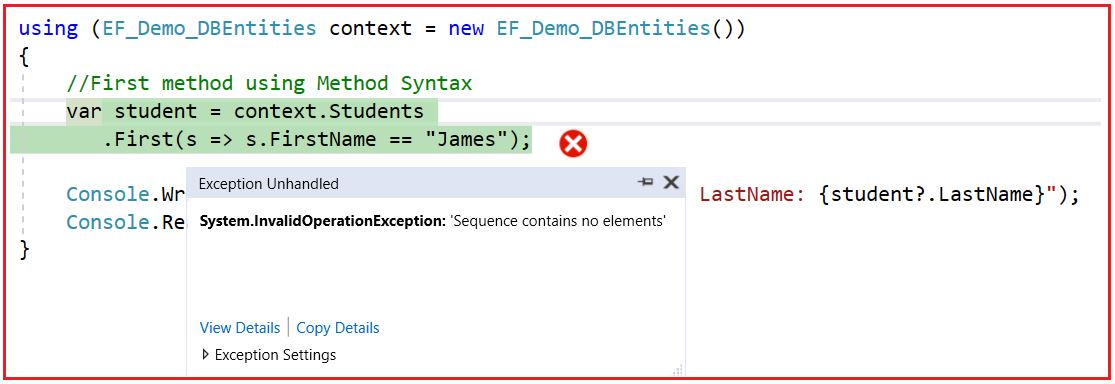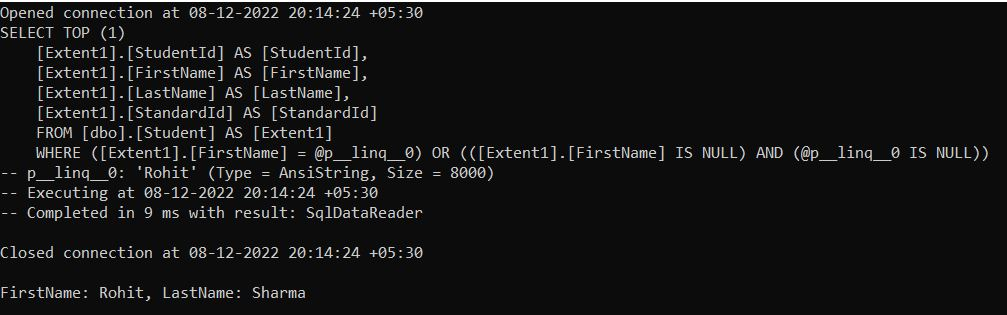# LINQ to Entities Queries in Entity Framework

## LINQ to Entities Queries in Entity Framework

In this article, I am going to discuss LINQ to Entities Queries in Entity Framework. Please read our previous article where we discussed Different Approaches to Querying in Entity Framework. We are going to work with the same example that we created in our Introduction to Entity Framework Database First article. Please read our introduction to Entity Framework Database First article before proceeding to this article.

##### LINQ-to-Entities Queries

In this article, I will show you how to write LINQ-to-Entities queries and get the result in Entity Framework. Please visit our LINQ Tutorials to learn LINQ step by step in detail.

The Entity Framework DbSet class is derived from the IQuerayable interface. As the DbSet class is derived from the IQuerayable interface, so, we can use LINQ (Language Integrated Query) for querying against DbSet and the DbSet then covert the queries into the SQL queries that the underlying database can understand and execute and gets the result set, converts it into appropriate entity type objects and returns it as a query result.

##### What is LINQ?

The LINQ (Language Integrated Query) is a part of a language but not a complete language. It was introduced by Microsoft with .NET Framework 3.5 and C# 3.0 and is available in System.Linq namespace.

LINQ provides us with a common query syntax that allows us to query the data from various data sources. That means using a single query we can get or set the data from various data sources such as SQL Server database, XML documents, ADO.NET Datasets, and any other in-memory objects such as Collections, Generics, etc. For a better understanding of how LINQ works please read out Architecture of LINQ article.

##### What is Projection?

Projection is nothing but the mechanism which is used to select the data from a data source. You can select the data in the same form (i.e. the original data in its original state). It is also possible to create a new form of data by performing some operations on it. For example, let’s assume in the database table, there is a Salary column. If you want you can get the Salary Column Values as it is i.e. in their original form. But, if you want to modify the Salary column values by adding a bonus amount of 10000, then it is also possible. If this is not clear at the moment, then don’t worry, we will understand this with real-time examples.

##### LINQ Query Operators:

The LINQ Standard query methods are implemented as Extension Methods and those methods can be used with LINQ-to-Entities queries. The following are examples of some of the standard LINQ Query methods.

1. First() or FirstOrDefault()
2. Single()or SingleOrDefault()
3. ToList()
4. Count()
5. Min() and Max()
6. Sum()
7. Distinct()
8. Last() or LastOrDefault()
9. Average(), and many more

Note: In addition to the above LINQ Extension methods, you can use the DbSet Find() method to search an entity based on the primary key value.

##### DbSet Find() Method in Entity Framework:

The Find method belongs to the DbSet class. This method is used to Find an entity with the given primary key values. If an entity with the given primary key values exists in the context, then it is returned immediately without making a request to the database. Otherwise, a request is made to the database for an entity with the given primary key values and this entity, if found, is attached to the context and returned. If no entity is found either in the context or in the database, then null is returned.

##### Example to Understand DbSet Find() Method in Entity Framework:

In our example, EF_Demo_DBEntities is our DbContext class and Students is the DbSet property and we want to find the student whose StudentId is 1. Here, StudentId is the primary key in our Student table.

```using System;
namespace DBFirstApproach
{
class Program
{
static void Main(string[] args)
{
using (EF_Demo_DBEntities context = new EF_Demo_DBEntities())
{
var student = context.Students.Find(1);
Console.WriteLine(\$"FirstName: {student?.FirstName}, LastName: {student?.LastName}");
}
}
}
}
```
##### First or FirstOrDefault Method in Entity Framework

These two methods are used when you want to fetch a single entity from the list of entities. For example, it might be possible that there is more than one student with the FirstName Rohit, but our requirement is only to fetch the first student whose name is Rohit. In this case, we need to use either the First or FirstOrDefault method as shown in the below example. Again, we can write the LINQ queries in two different ways i.e. using Method Syntax and using Query Syntax,

###### LINQ Method Syntax:
```using System;
using System.Linq;

namespace DBFirstApproach
{
class Program
{
static void Main(string[] args)
{
using (EF_Demo_DBEntities context = new EF_Demo_DBEntities())
{
//FirstOrDefault method using Method Syntax
var student1 = context.Students
.FirstOrDefault(s => s.FirstName == "Rohit");
Console.WriteLine(\$"FirstName: {student1?.FirstName}, LastName: {student1?.LastName}");

//First method using Method Syntax
var student2 = context.Students
.First(s => s.FirstName == "Rohit");

Console.WriteLine(\$"FirstName: {student2?.FirstName}, LastName: {student2?.LastName}");
}
}
}
}
```
###### Output:###### LINQ Query Syntax:
```using System;
using System.Linq;
namespace DBFirstApproach
{
class Program
{
static void Main(string[] args)
{
using (EF_Demo_DBEntities context = new EF_Demo_DBEntities())
{
//FirstOrDefault method using Query Syntax
var student1 = (from s in context.Students
where s.FirstName == "Rohit"
select s).FirstOrDefault();
Console.WriteLine(\$"FirstName: {student1?.FirstName}, LastName: {student1?.LastName}");

//First method using Query Syntax
var student2 = (from s in context.Students
where s.FirstName == "Rohit"
select s).First(); ;

Console.WriteLine(\$"FirstName: {student2?.FirstName}, LastName: {student2?.LastName}");
}
}
}
}
```

Here, you will also get the same output as the previous example.

Note: Both First and FirstOrDefault methods in LINQ are used to return the first element from a data source. But if the data source is null or if the specified condition does not return any data, then the First method will throw an exception while the FirstOrDefault method will not throw an exception instead it returns a default value based on the data type of the element.

In our database, the Student table does not have any student with the name James and if we try to fetch the student whose FirstName is James using the LINQ First method, then it will throw an exception as shown in the below example.

```using System;
using System.Linq;

namespace DBFirstApproach
{
class Program
{
static void Main(string[] args)
{
using (EF_Demo_DBEntities context = new EF_Demo_DBEntities())
{
//First method using Method Syntax
var student = context.Students
.First(s => s.FirstName == "James");

Console.WriteLine(\$"FirstName: {student?.FirstName}, LastName: {student?.LastName}");
}
}
}
}
```
###### Output:##### Parameterized Query in Entity Framework:

The Entity Framework builds and executes a parameterized query in the database if the LINQ-to-Entities query uses parameters. In the following example, we use the parameter FirstName.

```using System;
using System.Linq;
namespace DBFirstApproach
{
class Program
{
static void Main(string[] args)
{
using (EF_Demo_DBEntities context = new EF_Demo_DBEntities())
{
//The following statement will log the generated statement in the Console window
context.Database.Log = Console.Write;

//Creating a Variable
string firstName = "Rohit";

//Using the Variable
var student = context.Students
.FirstOrDefault(s => s.FirstName == firstName);

Console.WriteLine(\$"\nFirstName: {student?.FirstName}, LastName: {student?.LastName}");
}
}
}
}
```

Now, run the above code and you should get the following output. Please observe the generated SELECT SQL Statement and you can see it is using the parameter p__linq__0.##### ToList Method:

The ToList Method is used to create a System.Collections.Generic.List<T> collection from a System.Collections.Generic.IEnumerable<T>. This method causes the query to be executed immediately. For example, if you want to list all the students in a collection of type List, then you need to use the ToList() method as shown in the below example.

```using System;
using System.Linq;
namespace DBFirstApproach
{
class Program
{
static void Main(string[] args)
{
using (EF_Demo_DBEntities context = new EF_Demo_DBEntities())
{
var studentList = context.Students.Where(x => x.StandardId == 1).ToList();
foreach(var student in studentList)
{
Console.WriteLine(\$"FirstName: {student?.FirstName}, LastName: {student?.LastName}");
}

}
}
}
}
```

Note: Along with ToList, you can also use ToArray, ToDictionary or ToLookup as per your business need.

##### GroupBy Method:

The group by operator or GroupBy extension method exactly does the same thing as the Group By clause does in SQL Query. This method takes a flat sequence of elements and then organizes the elements into groups (i.e. IGrouping<TKey, TSource>) based on a given key. The following example gets the results grouped by each Standard.

```using System;
using System.Linq;
namespace DBFirstApproach
{
class Program
{
static void Main(string[] args)
{
using (EF_Demo_DBEntities context = new EF_Demo_DBEntities())
{
//GroupBy using Method syntax
Console.WriteLine("GroupBy using Method syntax");
var studentsMS = context.Students.GroupBy(s => s.StandardId);

foreach (var groupItem in studentsMS)
{
Console.WriteLine(\$"StandardId : {groupItem.Key}");
foreach (var student in groupItem)
{
Console.WriteLine(\$"\tFirstName: {student?.FirstName}, LastName: {student?.LastName}");
}
}

Console.WriteLine();

//GroupBy using Query syntax
Console.WriteLine("GroupBy using Query syntax");
var studentsQS = from s in context.Students
group s by s.StandardId into studentsByStandard
select studentsByStandard;

foreach (var groupItem in studentsQS)
{
Console.WriteLine(\$"StandardId : {groupItem.Key}");
foreach (var student in groupItem)
{
Console.WriteLine(\$"\tFirstName: {student?.FirstName}, LastName: {student?.LastName}");
}

}

}
}
}
}
```

When you execute the above program, the following SQL Query will execute in the database for both Method and Query Syntax. We captured this query using the SQL Profiler tool.

```SELECT
[Project2].[C1] AS [C1],
[Project2].[StandardId] AS [StandardId],
[Project2].[C2] AS [C2],
[Project2].[StudentId] AS [StudentId],
[Project2].[FirstName] AS [FirstName],
[Project2].[LastName] AS [LastName],
[Project2].[StandardId1] AS [StandardId1]
FROM ( SELECT
[Distinct1].[StandardId] AS [StandardId],
1 AS [C1],
[Extent2].[StudentId] AS [StudentId],
[Extent2].[FirstName] AS [FirstName],
[Extent2].[LastName] AS [LastName],
[Extent2].[StandardId] AS [StandardId1],
CASE WHEN ([Extent2].[StudentId] IS NULL) THEN CAST(NULL AS int) ELSE 1 END AS [C2]
FROM   (SELECT DISTINCT
[Extent1].[StandardId] AS [StandardId]
FROM [dbo].[Student] AS [Extent1] ) AS [Distinct1]
LEFT OUTER JOIN [dbo].[Student] AS [Extent2] ON ([Distinct1].[StandardId] = [Extent2].[StandardId]) OR (([Distinct1].[StandardId] IS NULL) AND ([Extent2].[StandardId] IS NULL))
)  AS [Project2]
ORDER BY [Project2].[StandardId] ASC, [Project2].[C2] ASC
```
##### OrderBy Method:

We use the OrderBy operator with ascending/descending keywords in the LINQ query to get the sorted entity list. In simple terms, we can say that Ordering is nothing but a process to manage the data in a particular order. It is not changing the data or output rather this operation arranges the data in a particular order i.e. either ascending order or descending order. In this case, the count is going to be the same but the order of the element is going to change.

In the following example, we sorted the students based on the FirstName. Here, I am showing you how to use Order By using both the Method and Query syntax.

```using System;
using System.Linq;
namespace DBFirstApproach
{
class Program
{
static void Main(string[] args)
{
using (EF_Demo_DBEntities context = new EF_Demo_DBEntities())
{
//Order By using Query syntax
var studentsQS = from s in context.Students
orderby s.FirstName ascending
select s;

//Order By using Method syntax
var studentsMS = context.Students.OrderBy(s => s.FirstName).ToList();

foreach (var student in studentsQS)
{
Console.WriteLine(\$"\tFirstName: {student?.FirstName}, LastName: {student?.LastName}");
}

}
}
}
}
```

When you execute the above program, the following SQL Script is created and executed in the database for both Method and Query syntax.

```SELECT
[Extent1].[StudentId] AS [StudentId],
[Extent1].[FirstName] AS [FirstName],
[Extent1].[LastName] AS [LastName],
[Extent1].[StandardId] AS [StandardId]
FROM [dbo].[Student] AS [Extent1]
ORDER BY [Extent1].[FirstName] ASC
```
##### Anonymous Object Result:

Instead of projecting the data to a particular type like Student, we can also project the data to an anonymous type using LINQ-to-Entities queries. Here, you may choose some of the properties or all of the properties of an entity as a result. The following example returns a list of anonymous objects which contain only the FirstName and LastName properties. The projection Result in the below example will be an anonymous type because there is no class/entity which has these properties. So, the compiler will mark it as anonymous.

```using System;
using System.Linq;
namespace DBFirstApproach
{
class Program
{
static void Main(string[] args)
{
using (EF_Demo_DBEntities context = new EF_Demo_DBEntities())
{
//Query Syntax
var selectQuery = (from std in context.Students
select new
{
firstName = std.FirstName,
lastName = std.LastName
});

foreach (var student in selectQuery)
{
Console.WriteLine(\$"FirstName: {student?.firstName}, LastName: {student?.lastName}");
}

//Method Syntax
var selectMethod = context.Students.
Select(std => new
{
firstName = std.FirstName,
lastName = std.LastName
}).ToList();
foreach (var student in selectMethod)
{
Console.WriteLine(\$"FirstName: {student?.firstName}, LastName: {student?.lastName}");
}

}
}
}
}
```

When you execute the above program, the following SQL Script is executed in the database for both Method and Query syntax.

```SELECT
1 AS [C1],
[Extent1].[FirstName] AS [FirstName],
[Extent1].[LastName] AS [LastName]
FROM [dbo].[Student] AS [Extent1]
```
##### Join Method:

The LINQ Inner join is used to return only the matching records from both the data sources while the non-matching elements are removed from the result set. So, if you have two data sources let us say Student and StudentAddress, and when you perform the LINQ inner join, then only the matching elements i.e. the records which exist in both Student and StudentAddress table are included in the result set. For better understanding, please have a look at the following example.

```using System;
using System.Linq;
namespace DBFirstApproach
{
class Program
{
static void Main(string[] args)
{
using (EF_Demo_DBEntities context = new EF_Demo_DBEntities())
{
var JoinUsingMS = context.Students //Outer Data Source
.Join(
student => student.StudentId, //Inner Key Selector
(student, studentaddress) => new //Projecting the data into an anonymous type
{
StudentName = student.FirstName +" "+ student.LastName,
}).ToList();

foreach (var student in JoinUsingMS)
{
}

var JoinUsingQS = (from student in context.Students
select new
{
StudentName = student.FirstName + " " + student.LastName,
}).ToList();

foreach (var student in JoinUsingQS)
{
}

}
}
}
}
```

When you execute the above program, the following SQL Script is executed in the database for both Method and Query Syntax.

```SELECT
[Extent1].[StudentId] AS [StudentId],
CASE WHEN ([Extent1].[FirstName] IS NULL) THEN N'' ELSE [Extent1].[FirstName] END + N' ' + CASE WHEN ([Extent1].[LastName] IS NULL) THEN N'' ELSE [Extent1].[LastName] END AS [C1],